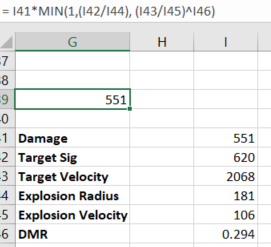# Missile math

Quick question for the math addicts here. Using EVE uni’s formula…

Damage=D×min(1,SE,(SVeEVt)drf)

I get…However using pyfa graph I get 119.3 DPS for the same profiles. Is there something pfya takes into account that the raw missile formula does not?

Since 1 is one of the options, there is no way that `min(1,SE,(SVeEVt)drf)` may be greater than one. Use two MIN functions or read your spreadsheet’s docs to understand why it’s not choosing the right option.
edit: nvm i read it as 141* …

guessing that your missing piece is `DPS = damage / cycle time`

Ah I found part of the problem was missing two whole damn variables but still not matching pfya. Remember that the equation is dmg * the min statement

on the right is the 3 parts of the min statement individuallyDPS is just a placement number for the damage in the fomula. If you input volley and divide by cycle time after the fact the numbers stays the same.

1 Like

Based on only the velocities, i’m surprised you even get the 197dps.

Base on what i read the target ship would be out of the explosion radius before the explosion wave hits it ( based on explosion velocity ), even taking into account the 620m ship signature.

Missile Damage Calulation

The equation for missile damage is as follows:

Damage=D×min(1,SE,(SVeEVt)drf)

Here are the terms in the equation. Except for the “damage reduction factor”, these are the same as were defined in the Basic Picture section, above :

S signature radius of target Note: The bigger the better
Vt velocity of target Note: The smaller the better
D base damage of missile Note: The bigger the better
E explosion radius of missile Note: The smaller the better
Ve explosion velocity of missile Note: The bigger the better
drf damage reduction factor Note: The smaller the better
1 Like

Yeah so ran so in game tests and honestly have no clue wtf the actual equations are for it. The damage was consistent in all runs, but didn’t match the equations in the slightest and was actually a lot lower than even pyfa (yes taking into account resists). shrug Was able to confirm that heavy missiles are indeed still hot garbage without significant support.

Your DRF is wrong. For heavy missiles it is 0.682, not 0.294, according to EVE uni.

Yip, DPS only show full damage (taking into account ROF and reloading, but no flight-time or target size/velocity effects)

Running damage mods and ROF mods will increase max possible damage/dps.

Only Target signature mods, target velocity mods, explosion radius/velocity mods with help maintain/obtain that possible max damage/dps before resistance effects.

So scripts for missile precision increase explosion velocity and decrease explosion radius ( this helps to do better damage on small targets), Target Painter helps increase target sig, and webifiers, etc helps reduces target speed, these all helps missile exsplosion to fully hit target.

V = Velocity.
MIN = choose the lowest of all the numbers. In this case, it hard limits the maximum damage coefficient to 1.
log = natural logarithm of a number.
DRF = Damage Reduction Factor. These range from 2.6 to 5.5 last time I checked, which explains why it’s divided by log(5.5). This part of the formula is an exponent that curves the velocity reduction part of the formula.

Single missile applied damage = raw damage * MIN( 1 , SR/ER , ( (SR/ER) * (EV/V) ) ^ ( log(DRF) / log(5.5) ) )

Volley = applied damage * launchers.
DPS = volley / cycle time.

Essentially, there are two factors at work, the size ratio as determined by SR/ER, and the speed ratio. Assuming a DRF of 5.5, the exponent comes to 1, and it can be ignored for this, leaving us with just (SR/ER)*(EV/V), SR/ER, or 1, whichever is lowest. Let’s use a missile with 500 raw damage, and no skills or implants.

What a Target Painter does is increase the numerator (upper number) in the division. It just multiplies the size of the ship. So a 50% TP applied on a ship with sigRad of 100m would look like 150m to the formula. If your missile has 200m expRad, the size ratio goes from 100/200 = 0.5, to 150/200 = 0.75. Not bad.

A 50% Stasis Webifier on the other hand reduces the denominator of the velocity portion. This greatly changes things. 200/2000 = 0.1. If you apply that 50% web the velocity of the ship is cut in half from 2000m/s to 1000m/s. The coefficient jumps from 0.1 to 0.2 (200/1000). You just doubled your applied damage.

Remember that the MIN function considers size in addition to velocity, or just velocity, whichever is smallest. So in this situation with both the TP and web, you would get 0.75 * 0.2 = .15, or 15% application from your 500 damage missile, which is a pathetic 75 damage.

The only missiles in the game that do ~500 raw damage are cruise missiles and torpedoes. But they have much worse application stats than I used here. Light Missiles on the other hand do about 100 damage. A more realistic number in this situation would be something in the neighborhood of 15 damage.

I was thinking the same thing!

Op, is this planning for an actual scenario or just trying to figure out the maths involved with missiles?

OP, if you’re still around monitoring this thread: you seem to be using Fury heavy missiles, judging from the expl radius of 181 (which is larger than that for t1/faction or Precision t2) . In that case the power factor (your I46 cell) should be 0.882. Damage then becomes 118.8.

This topic was automatically closed 90 days after the last reply. New replies are no longer allowed.